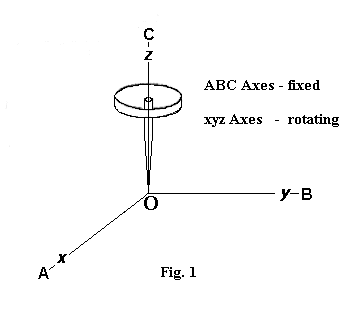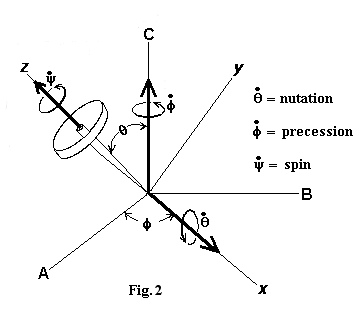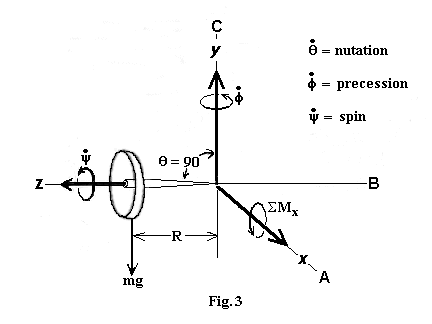MathsMore Maths## Derivation Of The Equations Of Gyroscopic Motion

### by Robert M. Beal

(May 2003)

The equations appearing in this document were taken from various sections of the textbook Engineering Mechanics - Statics and Dynamics, Third Edition, by R. C. Hibbeler (ISBN 0-02-354140-7), primarily from chapters 20 and 21 of the Dynamics section; if the reader wishes to delve deeper into a topic or needs clarification on a part of this document, I refer you to Hibbeler's textbook. I have pieced together the equations, with occasional modifications and my own comments, to (hopefully) provide a smoothly flowing derivation of the equations of gyroscopic motion starting from the most basic equation of dynamics, F = ma, and proceeding uninterrupted to the stated goal.

We will start with Newton's well-known and accepted equation relating force (F) to mass (m) and acceleration (a). For a particle, this is (bold type indicates a vector quantity):

SF = ma

This equation states that the sum of the external forces acting on a particle equals the particle's mass times its acceleration. Actually, Newton's original formulation related the external forces to the particle's linear momentum:

SF = mv'

Here, v is velocity, v' is the time rate of change of velocity (dv/dt) , and mv' is the time rate of change of linear momentum. If we chose a reference point O and r is a position vector to the particle, we can take the cross product of both sides of this equation to get an expression that relates the moment of the forces (Mo) acting on the particle to the angular momentum (Ho) of the particle with respect to the reference point O:

r x SF = r x mv'

Recognizing that r x SF is the Sum of the Moments ( SMo) of the forces acting on the particle about the reference point O, we can write:

SMo = r x mv'

Since the particle's angular momentum is Ho = r x mv, if we take the time derivative of angular momentum, we have:

d(Ho)/dt = d(r x mv)/dt
H'o = r' x mv + r x mv'

Recognizing that v = dr/dt = r' , we have:

H'o = r' x mr' + r x mv'

Since the cross product of two equivalent vectors is zero, r' x mr' = m(r' x r') = 0, therefore:

H'o = r x mv'

Substituting into the equation for the Sum of the Moments, we have:

SMo = H'o

That is, given a moving particle, the Sum of the Moments about a point O is equal to the time rate of change of the particle's angular momentum.

For a system of particles, we sum the moments of the forces of all the particles. In the following equation, Sf is the internal force acting on the ith particle due to all the other particles in the system:

Si[(r x SF)i + (r x Sf)i] = Si[H'o]i

The internal forces cancel out because corresponding pairs of internal forces are equal in magnitude and opposite in direction, therefore, Si(r x Sf)i = 0, and the resultant equation for a system of particles has the same form as the equation for a single particle:

Si[r x SF]i = Si[H'o]i
SMo = H'o

In words, this states that the Sum of the Moments about point O due to the external forces acting on a system of particles is equal to the time rate of change of the angular momentum of the system of particles about this same reference point O.

We recognize that any solid body is a system of particles, so this equation applies to the analysis of a gyroscope.

What we need now is an expression for the angular momentum Ho or its time derivative H'o that has attributes that we can physically measure such as mass, radius, angular velocity, and angular acceleration. If we consider a particle in the body having an incremental mass Dm and having an angular velocity w with respect to reference point O, since v = w x r, we can write:

[DHo]i = r x Dmivi
[DHo]i = [r x (w x r)]iDmi

Summing all the incremental angular momenta for all the particles in the body, we have:

Si[DHo]i = Si[r x (w x r)]iDmi

If we let D approach 0, then D[Ho]i and Dmi become differentials, and we can replace Si with integration. I will use z instead of the usual integral sign simply because I don't have the usual integral sign available:

zHodHo = zmr x (w x r) dm
Ho = zmr x (w x r) dm

If we place xyz reference coordinate axes at point O, we can define Ho, r, and w in terms of i, j, and k components as follows:

Ho = Hx i + Hy j + Hz k
r = x i + y j + z k
w = wx i + wy j + wz k

Substituting into the above integral expression for Ho, we have:

Hx i + Hy j + Hz k =
zm(x i + y j + z k) x [(wx i + wy j + wz k ) x (x i + y j + z k)]dm

Computing the cross products and combining terms gives:

Hx i + Hy j + Hz k =
[wxzm(y2+z2)dm - wyzmxy dm - wzzmxz dm] i
+ [- wxzmxy dm + wyzm(x2+z2)dm - wzzmyz dm] j
+ [- wxzmzx dm - wyzmyz dm + wzzm(x2+y2)dm] k

Recognizing that the above integrals are moments of inertia and products of inertia, we can write the above as the following scalar equations:

Hx = + Ixxwx - Ixywy - Ixzwz
Hy = - Iyxwx + Iyywy - Iyzwz
Hz = - Izxwx - Izywy + Izzwz

If we choose a coordinate system such that at least two of the three orthogonal planes defined by the coordinate system are axes of symmetry for the body, then all the products of inertia become zero, and our equations reduce to:

Hx = Ixxwx
Hy = Iyywy
Hz = Izzwz

If the axes are chosen as described above, they are called principal axes of inertia. We now have the angular momentum Ho in a useful form since the angular velocity can be measured and the moments of inertia (I), which depend only upon the mass and physical dimensions of the body, can be looked up in a table or calculated by hand.Our analysis of the gyroscope will be greatly simplified if we choose a coordinate system such that at least two of the three orthogonal planes defined by the coordinate system are axes of symmetry for the gyroscope. If the coordinate system is chosen to met this criteria, then all the products of inertia will become zero, and we only have to include moments of inertia in our calculations. To accomplish this, we will use a rotating coordinate system with origin at the pivot point of the gyro. The rotating coordinate system will follow the gyro's nutation and precession but not its spin. We will call the angular velocity of the rotating reference W = nutation + precession = q' + f' and label the rotating axes as the xyz axes. We will also have a fixed reference coordinate system which will also have its origin at the pivot point of the gyro and we will call this the ABC axes. The angular velocity of the gyro with respect to the fixed ABC axes will be w = nutation + precession + spin = q' + f' + y'(refer to Fig. 1 and Fig. 2). The angles q, f, and y are called Euler angles after the Swiss mathematician Leonhard Euler.

Since the xyz axes are rotating with respect to the fixed ABC axes, it is necessary to evaluate H'o taking into account that the unit vectors i, j, and k are not constants since they are changing direction. We then have:

(H'o)ABC = H'x i + H'y j + H'z k + Hx(di/dt) + Hy(dj/dt) + Hz(dk/dt)

where (H'o)ABC is the time derivative of the angular momentum with respect to the fixed ABC axes.
The time derivatives of the unit vectors can be expressed as:

di/dt = W x i
dj/dt = W x j
dk/dt = W x k

The Sum of the Moments equation can then be written as:

SMo = H'x i + H'y j + H'z k + W x Ho

SMx i + SMy j + SMz k = H'x i + H'y j + H'z k + W x Ho

The tables one finds for moments of inertia for bodies of various geometrical shapes are for the principal axes of inertia and are typically listed as Ixx, Iyy, and Izz. Since the center of gravity of the flywheel of our gyro lies at a distance R from the origin O of our coordinate axes, the x and y axes are not principal axes of inertia, but they are parallel to the principal axes of inertia of the flywheel. We can therefore use the Parallel Axis Theorem to relate the x and y components of the moments of inertia to the Ixx and Iyy moments of inertia as shown below. The Iz component does not present a problem since the z-axis passes thru the flywheel's center of gravity so it is a principal axis of inertia:

Ix = Ixx + mR2
Iy = Iyy + mR2
Iz = Izz

Keeping in mind the relationships between Ix, Iy, Iz and Ixx,Iyy, Izz, we can now express the angular momentum of the gyro as:

Hx = Ixwx
Hy = Iywy
Hz = Izwz

Substituting the above into the Sum of the Moments equation and taking the indicated time derivatives yields:

SMx i + SMy j + SMz k =

(Ixw'xi + Iyw'yj + Izw'zk) + [( Wxi + Wyj + Wzk) x (Ixwxi + Iywyj + Izwzk)]

Computing the cross products and combining terms, we get the following scalar equations for the Sum of the Moments:

SMx = Ixw'x - IyWzwy + IzWywz
SMy = Iyw'y - IzWxwz + IxWzwx
SMz = Izw'z - IxWywx + IyWxwy

Referring to Fig. 2, we can express the xyz components of the angular velocity W of the rotating axes and the xyz components of the angular velocity w of the gyroscope as follows:

W = q' + f'
W = Wx i + Wy j + Wz k
W = q' i + (f'sinq) j + (f'cosq) k

w = q' + f' + y'
w = wx i + wy j+ wz k
w = q' i + (f'sinq) j + (f'cosq + y' ) k

Substituting the components of W and w into the above scalar Sum of the Moments equations and computing the indicated time derivatives yields:

SMx = Ixq" - Iy(f')2cosqsinq + Izf'sinq(f'cosq + y')
SMy = Iy(f'q'cosq + f"sinq) - Izq'(f'cosq + y') + Ixf'q'cosq
SMz = Iz(- f'q'sinq + f"cosq + y") - Ixf'q'sinq + Iyf'q'sinq

In general, it would be very hard to arrive at a solution that satisfies these equations, however, in the specific case where the precession f' is constant, the spin y' is constant, and the nutation angle q = 900 is a constant 90 degrees as shown in Fig. 3 below, the solution becomes quite easy:In this case, we have:

q' = 0
f" = 0
y" = 0
cos(900) = 0
sin(900) = 1

and the Sum of the Moments equations reduce to:

SMx = Izf'y'
SMy = 0
SMz = 0

so the only moment in this case is the moment about the x-axis. There are no negative signs in this equation and we consistently used the right-hand-rule in expressing all vector quantities, therefore, all of the vectors - the sum of the moments about the x-axis (SMx), the precession of the gyro about the y-axis (f'), and the spin of the flywheel about the z-axis (y') all act along their respective positive axes as indicated in Fig. 3. Our analysis of the the gyroscope lying at 900 has boiled down to the following important result:

SMx = Izf'y'

If the only moment about the x-axis is the moment resulting from the weight of the flywheel (we are assuming the weight of the shaft is negligible), and if the flywheel is at a distance R from the pivot point (origin O), and recalling that Iz = Izz, we have:

mgR = Izzf'y'

where m is the mass of the flywheel and g is the acceleration due to gravity. This equation tells us that if the flywheel is lying at 900 at a distance R from the pivot point and the flywheel is spinning at a constant angular velocity y', then the gyro will not topple over as might be expected, but instead, it will precess about the positive y-axis at a constant angular velocity of f'.

For a solid circular disc, Izz = 1/2mr2 where r is the radius of the disc. If we instead concentrate most of the mass around the outer edge, then for a thin circular ring, Izz = mr2:

solid circular disk: mgR = 1/2mr2f'y'
thin circular ring: mgR = mr2f'y'

To counter the moment mgR, a gyro that uses a solid circular disk as the flywheel will precess twice as fast as a gyro that uses a thin circular ring (assuming both flywheels have the same mass m and radius r).

References:

Engineering Mechanics - Statics and Dynamics, Third Edition, by R. C. Hibbeler (ISBN 0-02-354140-7)

Engineering Mechanics - Dynamics by Anthony Bedford and Wallace Fowler (ISBN 0-201-58197-3)

Many thanks to Robert M. Beal (email: psisquared@Earthlink.net) for providing this document.